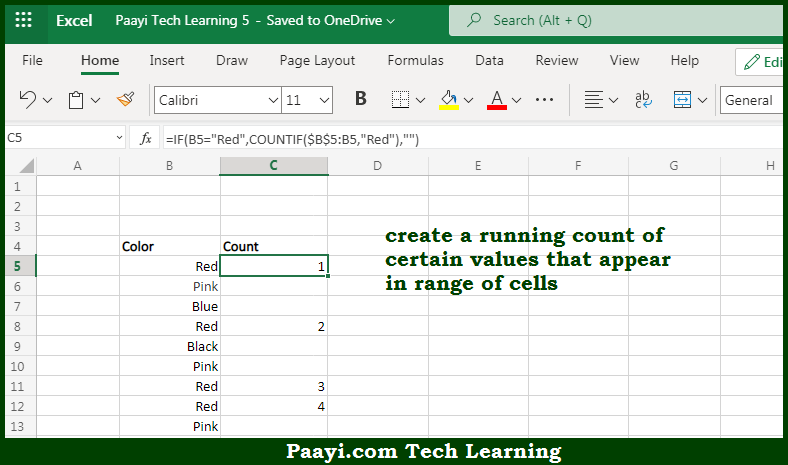# Learn How to Create Running COUNT of Occurrence in a List in Microsoft Excel

Written by | 0 Comments | 522 Views

In this article, you will learn how to COUNT various things in Microsoft Excel using a single or combination of functions and its purpose. You will also get to know how to Create Running COUNT of Occurrence in a List and see the generic formula.

Create Running COUNT of Occurrence in a List in Microsoft Excel

The main purpose of this formula is to create a running count of certain values that appear in the range of cells. Here we will learn how to perform running COUNT of occurrence in a list in Microsoft Excel. That implies, with the help of a formula based on the COUNTIF function and mixed expression you can able to create a running count of certain values that appear in the range of cells. So, with the help of this formula, you can able to perform a running COUNT of occurrence in a list.

General Formula to Create Running COUNT of Occurrence in a List

=COUNTIF(\$A\$1:A1,value)

The Explanation for the Create Running COUNT of Occurrence in a ListSo we know that with the help of the given formula above you can able to create a running count of certain values that appear in the range of cells. Here we will learn how to perform running COUNT of occurrence in a list in Microsoft Excel. As we know that when we copy the formula, the first cell in the reference is locked, but the second reference expands to include each new row. The COUNTIF function counts cells in the range on each row that are equal to "criteria", and the result is a running count. The outer layer of the formula uses the IF function to control when COUNTIF fires. So, with the help of this formula, you can able to create a running count of certain values that appear in the range of cells.